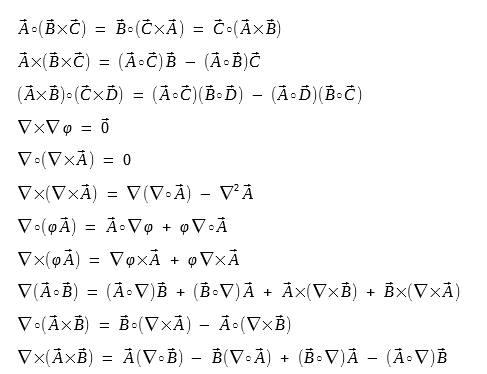# Vector Algebra

### Why Vector Algebra?

Most serious analysis of optical signal generation and propagation are based on Maxwell's Equations and boundary value problems such as optical modes and their propagation in optical fibers, waveguides, arrayed waveguide gratings (AWG), etc.

Maxwell's equations talk about the relationship of electric field intensity E, magnetic field intensity B,  the displacement current density D, and their gradient, divergence, curl operations. These quantities all have both magnitude and direction and are called vectors.

So one must have a solid understanding of vector analysis in order to fully understand and appreciate Maxwell's equations and optical signal propagations in optical fibers and waveguides.

### Vector Representations

In a three-dimensional Cartesian coordinate system, you only need to know the values of x, y, and z for each end of a vector, as shown below.Now consider the special case in which the vector is anchored to the origin of the coordinate system (that is, the tail is at the origin) as shown below. We can simply use the three coordinate numbers (x, y, z) of the end point (at the arrow), such as (5, 1, 3).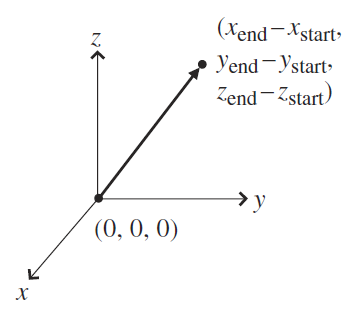The values are called the "components" of the vector, and the number of components is equal to the number of dimensions in the space in which the vector exists. So in a two-dimensional space a vector may be represented by a pair of numbers, and in four-dimensional spacetime vectors may appear as lists of four numbers.

### Unit Vectors

Unit vectors often serve as markers for various directions of interest and are also called "versors".

In the figure below, unit vectors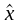,,(also called,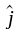,) point in the direction of the x-, y-, and z-axes of the three-dimensional Cartesian coordinate system.The length of unit vectors is exactly equal to one (also called unity). The unit is whatever the unit you are using for that axis (such as meter, degree, Hz, etc.).

Cartesian unit vectors,,can be drawn at any location, not just at the origin. So the Cartesian unit vectors show you the directions of the x, y, and z axes, not the location of the origin, as shown below.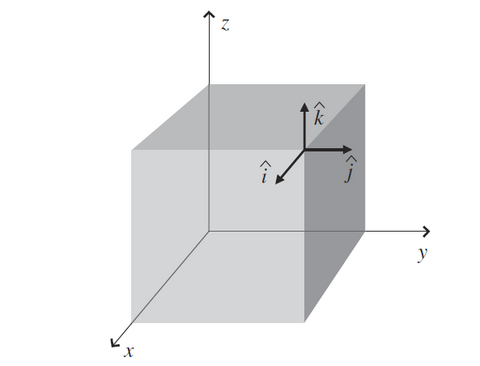Unit vectors are extremely useful at specifying the portion of a given vector pointing in a certain direction, since unit vectors don't have their own magnitude to pollute the calculation (which is exactly one).

### Vector Components

Vector components are the pieces used to make up the vector. Vectors can be written as a combination of vector components such as: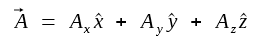where,,are the unit vectors,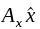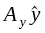are the x-, y-, and z-components of vector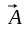, and Ax, Ay, Az are the magnitudes of vector components.

Two vectors A and B, which are not in the same direction nor in opposite directions, determine a plane. Their sum is another vector C in the same plane.

C = A + B can be obtained in three ways.

1. The parallelogram rule

The resultant C is the diagonal vector of the parallelogram formed by A and B drawn from the same point as shown below.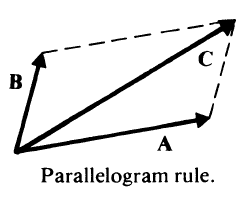The head of A connects to the tail of B. Their sum C is the vector drawn from the tail of A to the head of B, as shown below.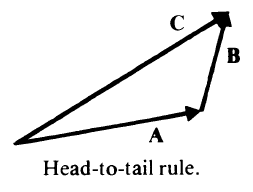The magnitude of C's vector component magnitudes (Cx, Cy, Cz) are the sum of the magnitudes of A (Ax, Ay, Az) and B (Bx, By, Bz). Thus

Cx = Ax + Bx
Cy = Ay + By
Cz = Az + Bz

The rationale for this approach is shown below.### Vector Subtraction

Vector subtraction can be defined in terms of vector addition in the following way.

A - B = A + (- B)

where - B is the negative of vector B; that is, - B has the same magnitude as B, but its direction is opposite to that of B. This is shown below.### Scalar or Dot Product

The dot product of two vectors is also called scalar product because the result is not a vector, but rather just a scalar quantity (which only has magnitude, but no direction).

The dot product between vectors A and B is defined as:where θ is the angle between A and B.

Or by Cartesian components: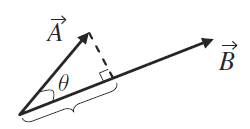The dot product A B represents the projection of A onto the direction of B multiplied by the length of B.

The result is exactly the same as the projection of B onto the direction of A. multiplied by the length of A. The order is irrelevant.

Applications:

1. The scalar product can be particularly useful when one of the vectors in the product is a unit vector. That's because the length of a unit vector is always one, so a scalar product such as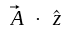finds the projection of vector A onto the direction of(the z-direction).

Thus to find the component of any vector in a given direction, you can simply form the dot product between that vector and the unit vector in the desired direction.

In physics and engineering problems, you frequently encounter the need to know the component of a vector A that's perpendicular to a specified surface. If you know the unit normal vector ()  for the surface, the scalar productgives you that perpendicular component of A.

2. The scalar product can be used to find the angle between two vectors. The derivation is: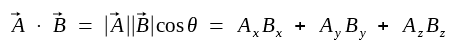Thus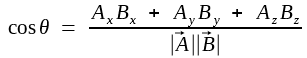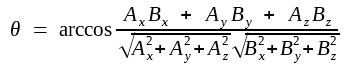3. The dot product between two perpendicular vectors gives a result of zero. (when θ = 90º, cosθ = 0). This feature is frequently used to determine whether two vectors are perpendicular to each other.

### Vector or Cross Product

Unlike dot product, the cross product (also called vector product) between two vectors results in another vector. This is very useful in physics such as when you are trying to find result of applying a force at the end of a lever arm or firing a charged particle into a magnetic field.

The vector or cross product of two vectors A and B, denoted A x B, is a vector perpendicular to the plane containing A and B; its magnitude is |A||B|sinθ , where θ is the smaller angle between A and B, and its direction follows that of the right hand rule when the fingers rotate from A to B through the angle θ.

This is shown below.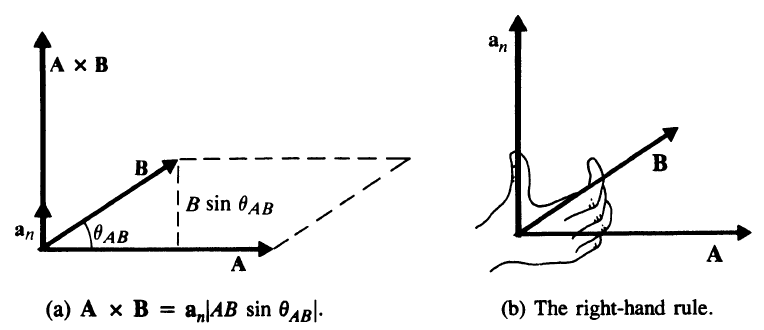Since |B|sinθ is the height of the parallelogram formed by the vectors A and B, we can see that the magnitude of A x B ( |A||B|sinθ) is always positive and is numerically equal to the area of the parallelogram.

A very important difference between the dot product and the cross product is that the order of the vectors is irrelevant for dot product but matters greatly for the cross product.

Cross product is not commutative (exchange positions), we find that:

B x A = - A x B

If you know the Cartesian components of both vectors, the cross product is given by: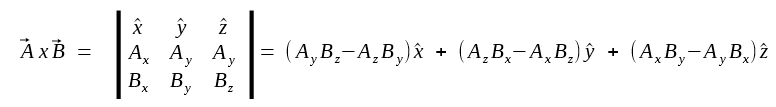Using the definition of the cross product and the right-hand rule, you can see that the following relations are true: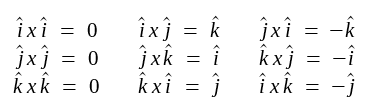Applications:

So if the angle θ between A and B is zero or 180º, which means A and B are parallel or antiparallel, the cross product between them is zero. This feature is frequent used to determine whether two vectors are parallel or antiparallel to each other.

Cross product is also used for torque problems and magnetic force problems.

### Product of Three Vectors

There are two kinds of products of three vectors; namely, the scalar triple product and the vector triple product

Scalar triple product:

Scalar triple product is defined as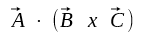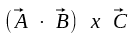has no meaning since (A B) gives a scalar, and you can't cross that scalar into C.

The operation is just a combination of dot and cross product so we can just write it down here as:The scalar triple product may be interpreted as the  volume of the parallelepiped formed by vectors A, B, and C.

This is shown below.In this figure, vectors A, B, and C represent the sides of a parallelepiped. The area of the base is |B x C|, and its height is equal to |A|cosφ, where φ is the angle between A and B x C.

Triple scalar product can be used to determine whether three vectors are coplanar (all three vectors lie in the same plane).

When A, B, C are in the sample plane, φ would be 90º and cosφ = 0, thus the height of the parallelepiped would be zero and the volume would be 0.

Vector triple product:

The vector triple product A x (B x C) are very useful when your engineering problem involves angular momentum and centripetal acceleration.

The triple vector product produces another vector. The triple vector product is somewhat tedious to calculate by brute force, but here is one quick expression:

A x (B x C) = B (A C) - C (A ∙ B)

Notice that on the right side, there are no circle or cross symbol between B and (A C), or between C and (A ∙ B). That is because (A ∙ B) and (A ∙ C) give result of a scalar quantity. So (A ∙ B) and (A ∙ C) are just scalar multipliers of vectors B and C.

A x (B x C) is not the same as (A x B) x C; the location of the parentheses matters greatly in the triple vector product.

So this means that the triple vector product is a vector and is a linear combination of the B and C (the second and third vectors). This is shown below.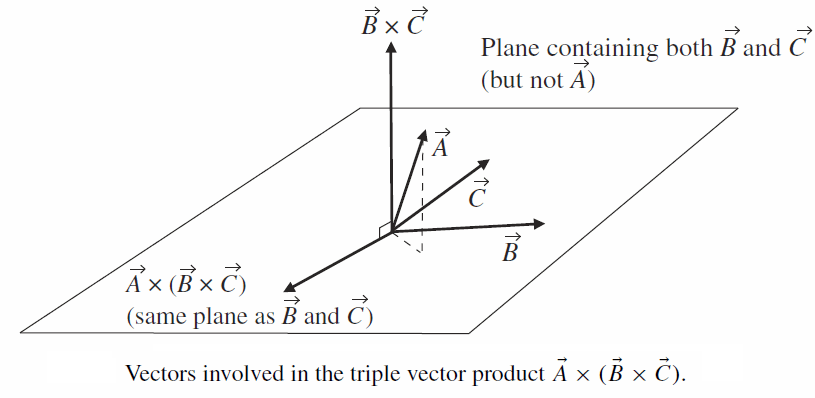### Vector Formulas